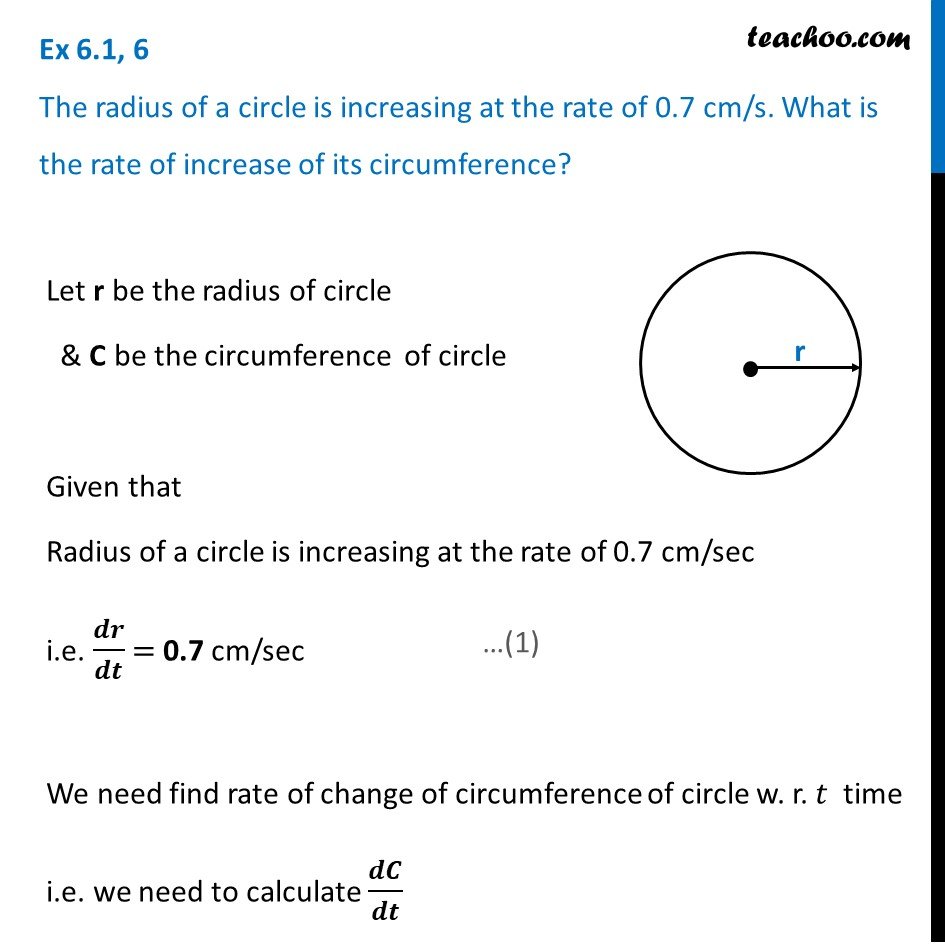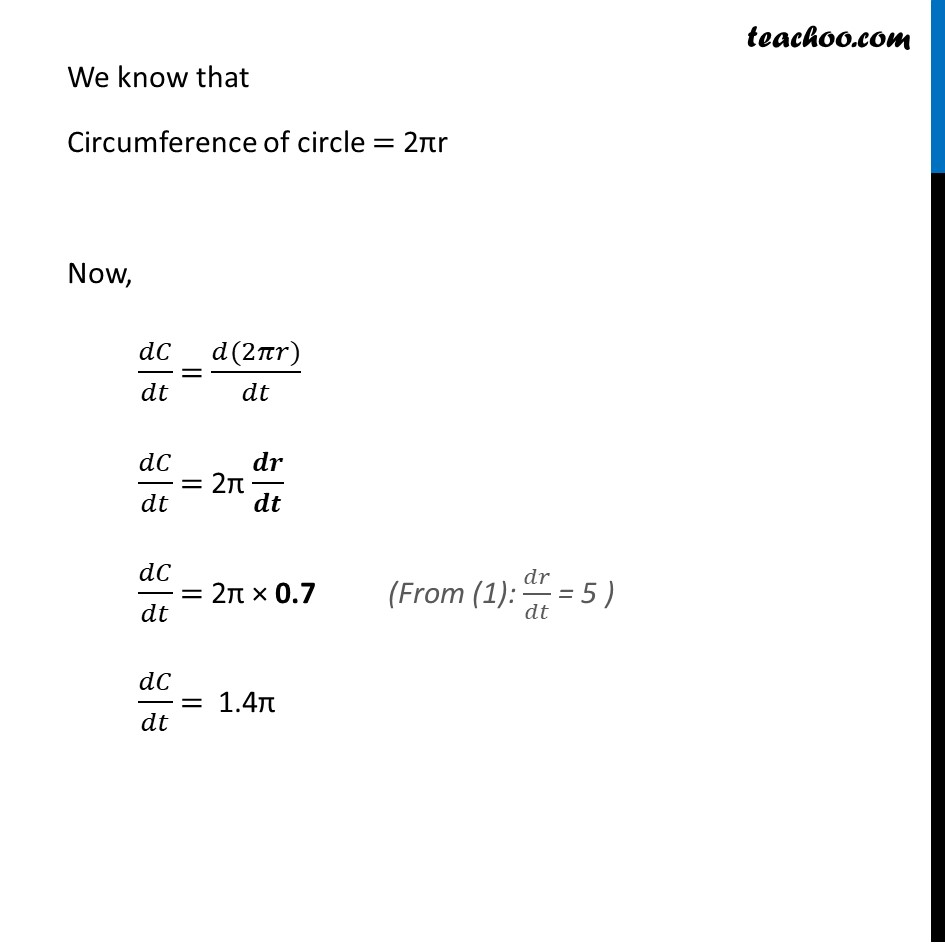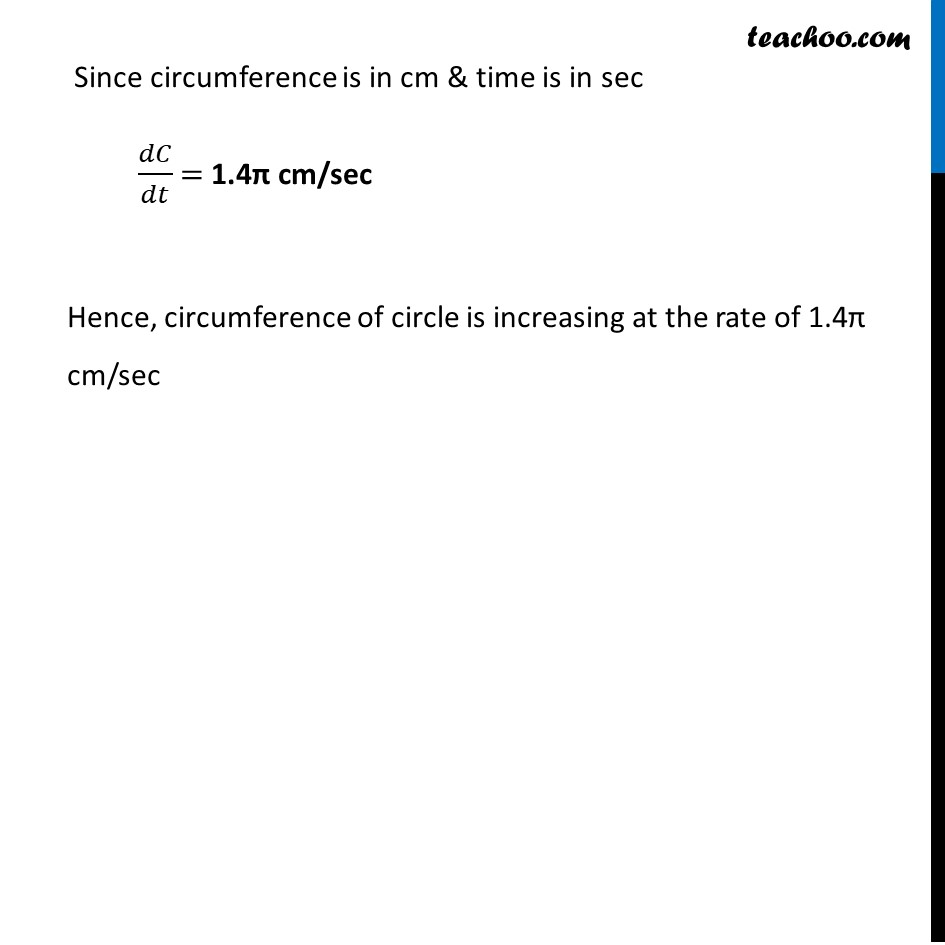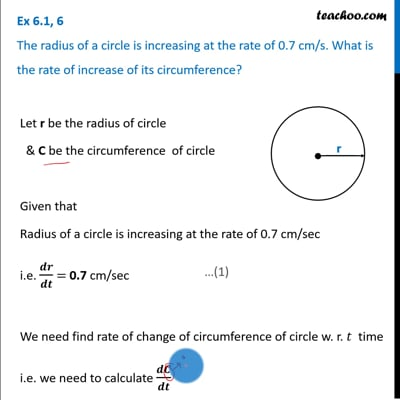Ex 6.1

Chapter 6 Class 12 Application of Derivatives
Serial order wiseThis video is only available for Teachoo black users

Solve all your doubts with Teachoo Black (new monthly pack available now!)

### Transcript

Ex 6.1, 6 The radius of a circle is increasing at the rate of 0.7 cm/s. What is the rate of increase of its circumference? Let r be the radius of circle & C be the circumference of circle Given that Radius of a circle is increasing at the rate of 0.7 cm/sec i.e. 𝒅𝒓/𝒅𝒕 = 0.7 cm/sec We need find rate of change of circumference of circle w. r. 𝑡 time i.e. we need to calculate 𝒅𝑪/𝒅𝒕 We know that Circumference of circle = 2πr Now, 𝑑𝐶/𝑑𝑡 = (𝑑(2𝜋𝑟))/𝑑𝑡 𝑑𝐶/𝑑𝑡 = 2π 𝒅𝒓/𝒅𝒕 𝑑𝐶/𝑑𝑡 = 2π × 0.7 𝑑𝐶/𝑑𝑡 = 1.4π (From (1): 𝑑𝑟/𝑑𝑡 = 5 ) Since circumference is in cm & time is in sec 𝑑𝐶/𝑑𝑡 = 1.4π cm/sec Hence, circumference of circle is increasing at the rate of 1.4π cm/sec